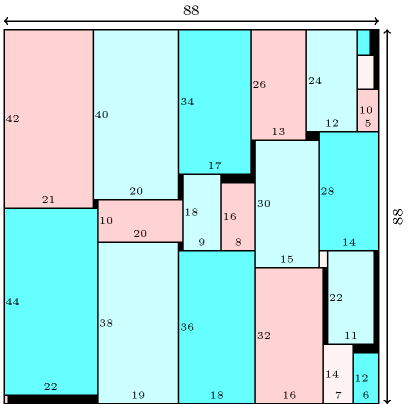### 3.7.234. Smallest square for packing consecutive dominoes

Find the smallest square $S$ where one can place $n$ rectangles of respective size $1×2,2×4,\cdots ,n×2·n$ so that they do not overlap and so that their borders are parallel to the borders of $S$. Each rectangle can be rotated by 90 degrees. The problem is described in http://www.stetson.edu/~efriedma/domino/. Figure 3.7.65 gives a solution for $n=22$ found by H. Simonis.

##### Figure 3.7.65. A solution to the smallest square for packing consecutive dominoes problem for $n=22$